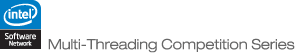JOINProblem Statement

Problem: CentrallyLocated

### Problem Statement

While travelling and living in space may be things of the future, there is no reason not to start planning now. Since the proverbial early bird gets the worm, we want an algorithm to figure out where the best locations will be to place gas stations in space. Naturally, to get the most business possible, we want the locations of our stores to be close to the houses of those living in space.

More formally, each house in space will be represented by its (x,y,z) coordinates. We are going to build k gas stations (select k (x,y,z) coordinates). We want to build the gas stations in such a way that the sum of the distances from the houses to the nearest gas station is minimized.

For example, if there were houses at (1,1,1), (2,2,2), (3,3,3), and (1,2,3), we might build two gas stations. One could be at (1.5,1.5,1.5) and the other at (2,2.5,3). The distance from each of the first two houses to the closest gas station (the one at (1.5,1.5,1.5)) is 0.87. The second two houses are closer to the other gas station, a distance of 1.12. The sum of these distances would be 3.97.

You will be given up to 500,000 points in space representing houses. You need to place up to 100 gas stations. Your score will be based on the sum of the distances as described, and your algorithm's runtime. For each test case, your improvement will be your average distance's improvement over the average distances that would be achieved by placing a single gas station at (500,500,500). Your score will be this improvement divided by the cube root of k and with a 1% penalty subtracted for every second of execution. Thus, if your average distance is 20 lower than the baseline average distance, k=8 and it takes you 4 seconds, your score will be 20/cuberoot(8)*0.96 = 9.6. To compute your final score, your scores on individual cases will simply be added up and divided by 100.

You are to write a method place, which takes x, y, z, and k. You should return a String[] with k elements, each element of which represents a gas station, formatted as "x y z".

The test cases will be generated as follows (unless otherwise stated, all random choices are uniform and independent and ranges are inclusive):
1. The number of points will be randomly chosen between 100 and 500,000.
2. A random integer is chosen between 1 and 100. This represents the number of cities.
3. A city center is chosen for each city. Each city center is placed by randomly choosing its x, y, and z coordinates between 0 and 1000.
4. For each city, a deviation is chosen between 10 and 200.
5. For each of the N points, it is associated with one of the cities at random.
6. Using the city center as the mean of a Gaussian distribution with the selected deviation, the point's location is generated.
7. k is chosen randomly between 2 and 100.

### Definition

 Class: CentrallyLocated Method: place Parameters: double[], double[], double[], int Returns: String[] Method signature: String[] place(double[] x, double[] y, double[] z, int k) (be sure your method is public)

### Notes

-The time limit for each test case is 50 seconds.
-All arithmetic will be done using doubles in Java. Strings will be converted to doubles via the Double.parseDouble function. Any format that works with that funcion is allowed.
-The minimum score for a test case is 0. If your solution would receive a negative score, you will get 0.
-While the city centers will have all their coordinates between 0 and 1000, the points themselves may not.
-There are 75 non-example test cases.
-The memory limit is 1GB, the thread limit is 32.
-A Gaussian distribution in three dimensions is just three independent Gaussian distributions, one in each coordinate. Thus to generate a point, each of its coordinates is generated independently.
-Download all the examples in one file.

### Examples

0)

`There are 100 points generated from 4 cities.  k = 61 `
1)

`There are 200 points generated from 91 cities.  k = 92 `
2)

`There are 452567 points generated from 32 cities.  k = 49 `
3)

`There are 349732 points generated from 69 cities.  k = 51 `
4)

`There are 473721 points generated from 55 cities.  k = 4 `
5)

`There are 199085 points generated from 32 cities.  k = 52 `
6)

`There are 9113 points generated from 18 cities.  k = 27 `
7)

`There are 483528 points generated from 3 cities.  k = 43 `
`There are 166691 points generated from 80 cities.  k = 62 `
`There are 396537 points generated from 46 cities.  k = 54 `